# GSEB Solutions Class 12 Statistics Part 2 Chapter 3 Normal Distribution Ex 3

Gujarat Board Statistics Class 12 GSEB Solutions Part 2 Chapter 3 Normal Distribution Ex 3 Textbook Exercise Questions and Answers.

## Gujarat Board Textbook Solutions Class 12 Statistics Part 2 Chapter 3 Normal Distribution Ex 3

Section A

Answer the following questions by selecting a correct option from the given options:

Question 1.
Which of the following is probability density function for normal variable X with mean µ and standard deviation σ?
(a) f(x) = $$\frac{1}{\sigma \sqrt{2 \pi}} e^{-\frac{1}{2}\left(\frac{x-\mu}{\sigma}\right)}$$, – ∞ < x < ∞
(b) f(x) = $$\frac{1}{\sigma \sqrt{2 \pi}} e^{-\left(\frac{x-\mu}{\sigma}\right)^{2}}$$ – ∞ < x < ∞
(c) f(x) = $$\frac{1}{\sigma \sqrt{2 \pi}} e^{-\frac{1}{2}\left(\frac{x-\mu}{\sigma}\right)^{2}}$$ – ∞ < x < ∞
(d) f(x) = $$\frac{1}{\sigma \sqrt{2 \pi}} e^{-\frac{1}{2}\left(\frac{x-\mu}{\sigma}\right)^{2}}$$, 0 ≤ x < ∞
(c) f(x) = $$\frac{1}{\sigma \sqrt{2 \pi}} e^{-\frac{1}{2}\left(\frac{x-\mu}{\sigma}\right)^{2}}$$ – ∞ < x < ∞Question 2.
For a normal variable X with mean µ and standard deviation σ, which of the following is standard normal variable Z for it?
(a) Z = $$\frac{x-\sigma}{\mu}$$
(b) Z = $$\frac{\sigma-x}{\mu}$$
(c) Z = $$\frac{\mu-x}{\sigma}$$
(d) Z = $$\frac{x-\mu}{\sigma}$$
(d) Z = $$\frac{x-\mu}{\sigma}$$

Question 3.
Which of the following is probability density function for standard normal variable?
(a) f(z) = $$e^{-\frac{1}{2} z^{2}}$$, – ∞< x< ∞
(b) f(z) = $$\frac{1}{\sqrt{2 \pi}} e^{-\frac{1}{2} z^{2}}$$, – ∞< z < ∞
(c) f(z) = $$\frac{1}{\sqrt{2 \pi}} e^{-\frac{1}{2} z^{2}}$$, 0 < z < ∞
(d) f(z) = $$\frac{1}{\sqrt{2 \pi}} e^{-z^{2}}$$, – ∞ < z < ∞
(b) f(z) = $$\frac{1}{\sqrt{2 \pi}} e^{-\frac{1}{2} z^{2}}$$, – ∞< z < ∞

Question 4.
Which of the following are mean and variance of standard normal variable ?
(a) Mean = 0, Variance = 1
(b) Mean = 1, Variance = 0
(c) Mean = 0, Variance = 0
(d) Mean = 1, Variance = 1
(a) Mean = 0, Variance = 1

Question 5.
What is the total area under normal curve among the following?
(a) – 1
(b) 0
(c) 1
(d) 0.5
(c) 1Question 6.
What is the area under the normal curve to the right hand side of perpendicular line at X = µ?
(a) 0
(b) 0.5
(c) 1
(d) – 0.5
(b) 0.5

Question 7.
In normal distribution, usually which limits include 99 % of the observations?
(a) µ + 1.96σ
(b) µ ± 2σ
(c) µ ± 3σ
(d) µ ± 2.575σ
(d) µ ± 2.575σ

Question 8.
In normal distribution, usually what percentage of the observations are included in the limits µ ± σ?
(a) 34.13 %
(b) 95.45 %
(c) 68.26 %
(d) 50%
(c) 68.26 %

Question 9.
Which of the following is approximate value of mean deviation for normal variable?
(a) $$\frac{4}{5}$$ σ
(b) $$\frac{4}{5}$$ μ
(c) $$\frac{2}{3}$$ σ
(d) $$\frac{2}{3}$$ μ
(a) $$\frac{4}{5}$$ σQuestion 10.
Which of the following is approximate value of quartile deviation for standard normal variable ?
(a) $$\frac{2}{3}$$ σ
(b) $$\frac{2}{3}$$
(c) $$\frac{4}{5}$$ σ
(d) $$\frac{4}{5}$$
(b) $$\frac{2}{3}$$

Question 11.
Mean and the first quartile for a normal distribution are 11 and 3 respectively. Which of the following is the value of the third quartile ?
(a) 8
(b) 14
(c) 19
(d) 10
(c) 19

Question 12.
For a normal distribution, approximate value of mean deviation is 20. Which of the following is the value of quartile deviation?
(a) $$\frac{25}{3}$$
(b) $$\frac{32}{3}$$
(c) 24
(d) $$\frac{50}{3}$$
(d) $$\frac{50}{3}$$

Question 13.
In usual notation of normal distribution, x = 25; µ = 20 and σ = 5, then which of the following is the value of standard normal variable ?
(a) 1
(b) – 1
(c) 4
(d) $$\frac{10}{3}$$
(a) 1Question 14.
Mean of a normal variable X is 50. If the value of Z-score is – 2.5 for x = 25, then which of the following is a variance of the distribution ?
(a) 10
(b) 100
(c) 50
(d) 25
(b) 100

Question 15.
If the distribution of normal variable is shown as N (20, 4), then which of the following intervals includes 99.73 % of observations ?
(a) (18, 22)
(b) (16, 24)
(c) (14, 26)
(d) (12, 28)
(c) (14, 26)

Section B

Answer the following questions in one sentence:

Question 1.
Give the values of the constants used in probability density function of normal variable.
In probability density function of normal variable the values of the constants are π = 3.1416 and e = 2.7183.

Question 2.
What is the probability that a continuous random variable takes definite value?
The probability that a continuous random variable takes definite value is zero (0).

Question 3.
What is the shape of normal curve ?
The shape of normal curve is completely bell-shaped.

Question 4.
What is the skewness of normal distribution ?
The skewness of normal distribution is zero.

Question 5.
“Standard score is independent of unit of measurement.” Is this statement true or false?
“Standard score is independent of unit of measurement.” This statement is true.Question 6.
For which value of standard normal variable, the standard normal curve is symmetric on both the sides?
For Z = 0, the standard normal curve is symmetric on both the sides.

Question 7.
Which value of normal variable divides the area of normal curve in two equal parts ?
The value of normal variable X = µ mean divides the area of normal curve in two equal parts.

Question 8.
What percentage of area is covered under the normal curve within the range µ – 2σ to µ + 2σ?
95.45 % of area is covered under the normal curve within the range µ – 2σ to µ + 2σ.

Question 9.
Mean of a normal distribution is 13.25 and its standard deviation is 10. Estimate the value of its third quartile.
Here, µ = 13.25; σ = 10
Now, Q3 = µ + 0.675 σ
∴ Q3 = 13.25 + 0.675(10)
= 13.25 + 6.75
= 20
Hence, Q3 = 20

Question 10.
For a normal distribution having mean 10 and standard deviation 6, estimate the value of quartile deviation.
Here, µ = 10; σ = 6

Estimated value of quartile deviation:
In normal distribution, Quartile deviation ≈ $$\frac{2}{3}$$ σ
Putting, σ = 6
Quartile deviation ≈ $$\frac{2}{3}$$ ≈ = 4
Hence, the estimated value of quartile deviation obtained is 4.Question 11.
The approximate value of mean deviation for a normal distribution is 8. Find the value of its standard deviation.
Here, mean deviation = 8
In normal distribution, mean deviation ≈ $$\frac{4}{5}$$ σ
∴ 8 ≈ $$\frac{4}{5}$$ σ
∴ σ ≈ $$\frac{8 \times 5}{4}$$ ≈ 10
Hence, the standard deviation obtained is 10.

Question 12.
For a normal distribution, the estimated value of quartile deviation is 12. Find the value of its standard deviation.
Here, quartile deviation = 12
In normal distribution,
Estimated value of quartile deviation ≈ $$\frac{2}{3}$$ σ
∴ 12 ≈ $$\frac{2}{3}$$ σ
∴ σ ≈ $$\frac{12 \times 3}{2}$$ ≈ 18
Hence, the value of standard deviation obtained is 18.

Question 13.
For a probability distribution of standard normal variable, state the estimated limits for the middle 50 % observations.
For a probability distribution of standard normal variable, the estimated limits for the middle 50% observations is (Q1, Q3) = (- 0.675, 0.675).

Question 14.
The extreme quartiles of normal distribution are 20 and 30. Find its mean.
Here, Q1 = 20; Q3 = 30
∴ M = $$\frac{Q_{3}+Q_{1}}{2}$$ = $$\frac{30+20}{2}$$ = $$\frac{50}{2}$$ = 25
In normal distribution, Mean = Median = Mode
∴ Mean µ = 25Question 15.
The monthly expense of a group of persons follows normal distribution with mean ₹ 10,000 and standard deviation ₹ 1000. A student has obtained a Z-score = 1 for randomly selected person having monthly expense more than 11,000. Is this calculation of Z-score true? Give reason.
Here, µ = 10,000; σ = 1000
For x > 11000, Z > $$\frac{x-\mu}{\sigma}$$ > $$\frac{11000-10000}{1000}$$ > 1
A student has obtained a Z-score = 1. Hence, this calculation of Z-score is not true.
Reason: For given data, we must have Z ≥ 1.

Question 16.
The age of a group of persons follows normal distribution with mean 45 years and standard deviation 10 years. Calculate Z-score for a randomly selected person having age 60 years.
Here, µ = 45; σ = 10, x – 60
∴ Z = $$\frac{x-\mu}{\sigma}$$ = $$\frac{60-45}{10}$$ = 1.5
Hence, Z-score = 1.5

Question 17.
Marks obtained by students of a school in Economics subject follows normal distribution with mean µ and standard deviation σ. The value of standard score that a randomly selected student obtained 60 marks is 1. If the variance of variable is 100 (marks)2, then find average marks.
Here, x = 60; Z = 1; σ2 = 100 ∴ σ = 10
∴ Z = $$\frac{x-\mu}{\sigma}$$
∴ 1 = $$\frac{60-\mu}{10}$$
∴ 10 = 60 – µ
∴ µ = 60 – 10
∴ µ = 50
Hence, the average marks obtained is 50.Section C

Answer the following questions as required:

Question 1.
Define probability density function of continuous random variable.
A function for obtaining probability that a continuous random variable assumes value between specified interval is called probability density function of continuous random variable. It is denoted by f(x).

Question 2.
Write the conditions for probability density function for continuous variable.
The conditions for probability density function for continuous variable are as follows:

• The probability that the value of random variable X lies within the specified interval must be non-negative (positive).
• The total probability that the random variable X assumes any value in the specified interval must be 1.

Question 3.
How is the normal curve drawn ?
The normal curve is drawn by considering different values of normal random variable X and its respective values of probability density function f(x).

Question 4.
Define probability density function for normal variable.
Probability density function for normal variable is defined as follows ;
f(x) = $$\frac{1}{\sigma \sqrt{2 \pi}} e^{-\frac{1}{2}\left(\frac{x-\mu}{\sigma}\right)^{2}}$$, – ∞ < X < ∞
Where, x = Value of normal variable X
µ = Mean of normal distribution
σ = Standard deviation of normal distribution
π = Constant = 3.1416
e = Constant = 2.7183Question 5.
What is the shape of standard normal curve ? To which value of variable it is symmetric ?
The shape of standard normal curve is complete bell-shaped. It is symmetric about the value of variable Z = 0.

Question 6.
Define standard normal variable and write its probability density function.
If X is normal variable with mean = µ and standard deviation = σ, then the random variable Z = $$\frac{\mathrm{X}-\mu}{\sigma}$$ is called the standard normal variable. It is free from units of measurement.

The probability density function of Z is as follows :
f(z) = $$\frac{1}{\sqrt{2 \pi}} e^{-\frac{1}{2} z^{2}}$$, – ∞ < z < ∞

Question 7.
A normal variable X has the probability density function as,
f(x) = constant × $$e^{-\frac{1}{50}(x-10)^{2}}$$, – ∞ < x < ∞
Find the first quartile from this information.
f(x) = constant × $$e^{-\frac{1}{50}(x-10)^{2}}$$, – ∞ < x < ∞
= constant × $$e^{-\frac{1}{2}\left(\frac{x-10}{5}\right)^{2}}$$
Hence, µ = 10, σ = 5

First Quartile Q1:
25 % of observations of the distribution is less than Q1.
∴ P [X ≤ Q1] = $$\frac{25}{100}$$ = 0.25
Now, z1 = $$\frac{\mathrm{Q}_{1}-\mu}{\sigma}$$ = $$\frac{Q_{1}-10}{5}$$P[Z ≤ z1] = 0.25
= P[- ∞ < Z ≤ 0] – P[z1 ≤ Z ≤ 0]
= P[0 ≤ Z < ∞] – P[0 ≤ Z ≤ z1]
∴ P[0 ≤ Z ≤ z1] = 0.5000 – 0.2500
= 0.2500
From the table, corresponding to probability 0.2486, the value of z1 = -0.67 and corresponding to probability 0.2518, the value of z1 = – 0.68
∴ For probability 0.25
z1 = $$\frac{(-0.67)+(-0.68)}{2}$$ = – 0.675
Now, z1 = $$\frac{Q_{1}-10}{5}$$
∴ – 0.675 = $$\frac{Q_{1}-10}{5}$$
∴ – 0.675 × 5 = Q1 – 10
∴ -3.375 = Q1 – 10
∴ 10 – 3.375 = Q1
∴ Q1 = 6.625 ≈ 6.63
Hence, first quartile obtained is 6.83.Question 8.
The extreme quartiles of a normal variable are 10 and 30. Find its mean deviation.
Here, Q1 = 10; Q3 = 30
∴ Quartile deviation = $$\frac{Q_{3}-Q_{1}}{2}$$ = $$\frac{30-10}{2}$$ = 10
Now, Quartile deviation = $$\frac{2}{3}$$ σ
∴10 = $$\frac{2}{3}$$ σ
∴σ = 10 × $$\frac{3}{2}$$ = 15
Mean deviation = $$\frac{4}{5}$$ σ
∴ Mean deviation = $$\frac{4}{5}$$ × 15 = 12
Hence, the mean deviation obtained is 12.

Question 9.
For a normal variable, mean deviation is 48 and its third quartile is 120. Estimate its first quartile.
Here, Mean deviation = 48; Q3 = 120
Now, mean deviation = $$\frac{4}{5}$$ σ
∴ 48 = $$\frac{4}{5}$$ σ
∴ c = 48 × $$\frac{4}{5}$$ ≈ 60
Quartile deviation ≈ $$\frac{2}{3}$$ σ
∴ Quartile deviation ≈ $$\frac{2}{3}$$ × 60
∴ $$\frac{Q_{3}-Q_{1}}{2}$$ ≈ 40
∴ Q3 – Q1 ≈ 80
Putting, Q3 = 120
120 – Q1 ≈ 80
∴ Q1 ≈ 120 – 80 ≈ 40
Hence, the first quartile obtained is 40.Question 10.
A normal variable X has the probability density function as,
f(x) = $$\frac{1}{10 \sqrt{2 \pi}} e^{-\frac{1}{2}\left(\frac{x-100}{10}\right)^{2}}$$, – ∞ < X < ∞
For this distribution, obtain the limits which include middle 68.26 % of the observations.
f (x) = $$\frac{1}{10 \sqrt{2 \pi}} e^{-\frac{1}{2}\left(\frac{x-100}{10}\right)^{2}}$$, – ∞ < X < ∞
∴ µ = 100, σ = 10
Now, the limits that include middle 68.26 % of the observations = µ ± σ
= (µ – σ) to (µ+ σ)
= (100 – 10) to (100 + 10)
= 90 to 110
Hence, the limits that include middle 68.26 % of the observations obtained is 90 to 110.

Question 11.
The probability that the value of standard normal variable lies between 0 and Z-score (z1) is 0.4950. Find the possible values of Z-score.
Here, P [0 ≤ Z ≤ z1) = 0.4950
From the table of area under the standard normal curve, corresponding to probability 0.4949 (which is near to 0.4950) the value of Z-score = 2.57. Also corresponding to probability 0.4951 (which is also near to 0.4950), the value of Z-score = 2.58. .
∴ Corresponding to probability 0.4950, the value of Z-score = $$\frac{2.57+2.58}{2}$$ = 2.575
Hence, the possible value of Z-score obtained is 2.575.Section D

Answer the following questions as required:

Question 1.
Define normal distribution and state the characteristics of normal curve.
The distribution of a normal variable X is called Normal distribution. It is denoted by N [µ, σ2).
The characteristics of normal curve:

• It is completely bell-shaped.
• It is asymptotic to X-axis, i.e., the tails of the curve never touch X-axis.
• The total area of the region bounded by normal curve and X-axis = Total probability 1.
• It is symmetric about the sides of mean µ of normal variate. So the area of the region of normal curve on both the sides of X = µ is equal to 0.5.
• P [µ ≤ X ≤ a] is the area of region bounded by X-axis and two perpendicular lines at X = µ and X = a.
• To find the area under the normal curve, the normal variable is transformed to standard normal variable Z and readymade table is used.

Question 2.
State the properties of normal distribution.
The normal distribution possesses the following properties:

1. The normal distribution is a probability distribution of continuous random variable.
2. µ and σ are its parameters.
3. The graph of density function of this distribution is continuous graph of bell-shape.
4. The normal curve is symmetric about X = µ.
5. The total area under normal curve is 1 and the area of the region of normal curve on both sides of X = µ is equal and 0.5.
6. The mean, median and mode of the distribution are equal, i.e., µ = M = M0.
7. The skewness of this distribution is zero.
8. The first quartile Q1 and third quartile Q3 are equidistant from the second quartile Q2 in this distribution, i.e., Q3 – Q2 = Q2 – Q1 and M = $$\frac{Q_{3}+Q_{1}}{2}$$
9. In the form of µ and σ the estimated values of Q1 and Q3 are obtained as follows:
Q1 ≈ µ – 0.675σ and Q3 ≈ µ + 0.675σ
10. Two tails of the curve of normal distribution are asymptotic to X-axis, i.e., they never touch X-axis.
11. In this distribution,
• Quartile deviation ≈ $$\frac{2}{3}$$σ
• Mean deviation ≈ $$\frac{4}{5}$$σ
12. The important areas under normal curve are as follows :
• The area under the normal curve between µ ± σ = 0.6826
• The area under the normal curve between µ ± 2σ= 0.9545
• The area under the normal curve between µ ± 3σ = 0.9973
• The area under the normal curve between µ ± 1.96σ = 0.95
• The area under the normal curve between µ ± 2.575σ = 0.99Question 3.
State the properties of standard normal distribution.
Some important properties of standard normal distribution are as follows :

1. It is a distribution of continuous random variable.
2. The curve of this distribution is completely bell-shaped.
3. The tails of the curve of this distribution are asymptotic to X-axis, i.e., they never touch X-axis.
4. The mean of this distribution is 0 (zero) and its variance and standard deviation is 1.
5. In this distribution the values of mean, median and mode are zero.
6. In this distribution the estimated values of Q1 and Q3 are as under :
Q1 ≈ – 0.675, Q3 ≈ 0.675
7. The curve of this distribution is completely symmetric about Z = 0 and its skewness is zero.
8. The area of the curve of this distribution bounded by X-axis and on both sides of Z = 0 is equal to 0.5.
9. In this distribution,
• Quartile deviation ≈ $$\frac{2}{3}$$
• Mean deviation ≈ $$\frac{4}{5}$$
10. The important areas related to standard normal curve are as follows :
• The area under the curve between z = ± 1 is 0.6826
11. The area under the curve between z = ± 2 is 0.9545
• The area under the curve between z = ± 3 is 0.9973
• The area under the curve between z = ± 1.96 is 0.95
• The area under the curve between z – ± 2.575 is 0.99

Question 4.
A normal distribution has mean 50 and variance 9. Find the probability that
(i) The value of normal variable X lies between 50 and 53.
(ii) The value of normal variable X lies between 47 and 53.
Here, µ = 50; σ2 = 9 ∴ σ = 3

(i) P [50 ≤ X ≤ 53]
For x1 = 50, z1 = $$\frac{x_{1}-\mu}{\sigma}$$ = $$\frac{50-50}{3}$$ = 0
For x2 = 53, z2 = $$\frac{x_{2}-\mu}{\sigma}$$ = $$\frac{53-50}{3}$$ = 1
∴ P [50 ≤ X ≤ 53] = P [0 ≤ Z ≤ 1] = 0.3413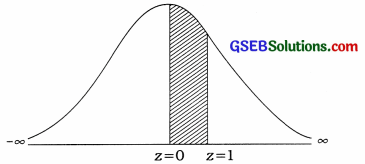(ii) P [47 ≤ X ≤ 53]
For x1 = 47, z1 = $$\frac{x_{1}-\mu}{\sigma}$$ = $$\frac{47-50}{3}$$ = $$\frac{-3}{3}$$ = – 1
For x2 = 53, z2 = $$\frac{x_{2}-\mu}{\sigma}$$ = $$\frac{53-50}{3}$$ = $$\frac{3}{3}$$ = 1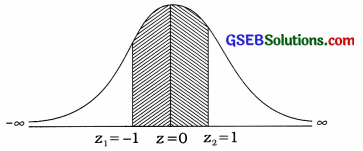∴ P [47 ≤ X ≤ 53] = P [- 1≤ Z ≤ 1]
= 2P[0 ≤ Z ≤ 1]
= 2 [0.3413]
= 0.6826Question 5.
If X is a normal variable with mean 100 and standard deviation 15, then find the percentage of observations
(i) Having valne more than 85.
(ii) Having value less than 130.
Here, µ = 100; σ = 15

(i) P [X ≥ 85]
For x = 85, z = $$\frac{x-\mu}{\sigma}$$ = $$\frac{85-100}{15}$$ = $$\frac{-15}{15}$$ = – 1
∴ P[X ≥ 85] = [Z ≥ – 1]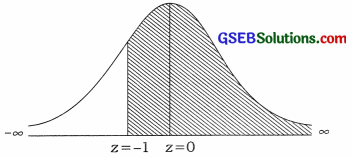Now,
P[z ≥ – 1] = P[-1 ≤ z ≤ 0] + P[0 ≤ z ≤ ∞]
= P[0 ≤ Z ≤ 1] + 0.5000
= 0.3413 + 0.5000 = 0.8413
Hence, the value of 0.8413 × 100 = 84.13 % observations is more than 85.

(ii) P [X ≤ 130]
For x = 130, z = $$\frac{x-\mu}{\sigma}$$ = $$\frac{130-100}{15}$$ = $$\frac{30}{15}$$ = 2
∴ P[X ≤ 85] = [Z ≤ 2]Now,
P[z ≤ 2] = P[- ∞ < Z ≤ 0] + P[0 ≤ z ≤ 2]
= 0.5000 + 0.4772 = 0.9772
Hence, the value of 0.9772 × 100 = 97.72 % observations is less than 130.Question 6.
The weight of randomly selected 500 adult persons from a region of a city follows normal distribution. The average weight of these persons is 55 n and its standard deviation is 7 kg.
(i) Estimate the number of persons ha ving weight between 41 kg to 62 kg.
(ii) Estimate the number of persons having weight less than 41 kg.
Here, N = 500; µ = 55; σ = 7

(i) P [41 ≤ X ≤ 62]
For x1 = 41, z1 = $$\frac{x_{1}-\mu}{\sigma}$$ = $$\frac{41-55}{7}$$ = $$\frac{-14}{7}$$ = – 2
For x2 = 62, z2 = $$\frac{x_{1}-\mu}{\sigma}$$ = $$\frac{62-55}{7}$$ = $$\frac{7}{7}$$ = 1
∴ P[41 ≤ X ≤ 62] = p[- 2 ≤ Z ≤ 1]Now, P [- 2 ≤ Z ≤ 1] = P[-2 ≤ Z ≤ 0] + P[0 ≤ Z ≤ 1]
= P[0 ≤ Z ≤ 2] + P[0 ≤ Z ≤ 1]
= 0.4772 + 0.3413
= 0.8185
Hence, the number of persons having weight between 41 kg to 62 kg
= N × P [- 2 ≤ Z ≤ 1]
= 500 × 0.8185
= 409

(ii) P [X ≤ 41]
For x = 41, z = $$\frac{x-\mu}{\sigma}$$ = $$\frac{- 14}{7}$$ = 2
∴ P [X ≤ 41 ] = P[Z ≤ – 2]Now, P [Z ≤ – 2] = P [- ∞ < Z ≤ – 2]
= P [- ∞ < Z ≤ 0] – P[- 2 ≤ Z ≤ 0]
= 0.5000 – P[0 ≤ Z ≤ 2]
= 0.5000 – 0.4772
= 0.0228
Hence, the number of persons having weight less than 41 kg
= N × P [Z ≤ – 2]
= 500 × 0.0228
= 11Question 7.
If probabilities for the value of standard normal variable Z are as under, then estimate the value of Z-score (Zj) :
(i) Area to the left of Z = z1 is 0.9928.
(ii) Area to the right of Z = z1 is 0.0250.
(i) Area to the left of Z = z1 is 0.9928.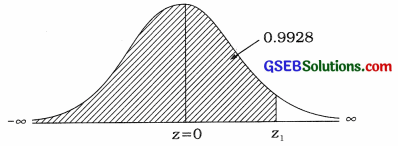P[Z ≤ z1] = 0.9928
∴ P[0 ≤ Z ≤ z1] = P[- ∞ < Z ≤ z1] – P [- ∞ < Z ≤ 0]
= 0.9928 – 0.5000
= 0.4928
From the table, for the probability 0.4927, z1 = 2.44 and for the probability 0.4929, z1 = 2.45.
For average probability = $$\frac{0.4927+0.4929}{2}$$
= 0.4928
z1 = $$\frac{2.44+2.45}{2}$$ = 2.445

(ii) Area to the right of Z = z1 is 0.0250.P [Z ≥ z1] = 0.0250
∴ P [0 ≤ Z ≤ z1] = 0.5000 – 0.0250
= 0.4750
From the table, for 0.4750, z1 = 1.96Question 8.
If Z is a standard normal variable then estimate the value of Z-score (zx) such that the following conditions are satisfied:
(i) Area to the left of Z = z1 is 0,15.
Area to the left of Z = z1 is 0.15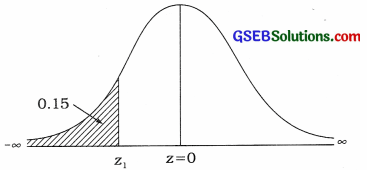P[Z ≤ z1] = 0.15
∴ P [z1 ≤ Z ≤ 0] = P [- ∞ < Z ≤ 0] – P [- ∞ < Z ≤ z1]
= 0.5000 – 0.15
= 0.3500
From the table, for area 0.3485, z1 = – 1.03 and for area 0.3508, z1 = – 1.04
∴ For area 0.3500,
z1 = $$\frac{(-1.03)+(-1.04)}{2}$$
= $$\frac{-2.07}{2}$$
= – 1.035

(ii) Area to the right of Z = z1 is 0.75.
Area to the right of Z = z1 is 0.75.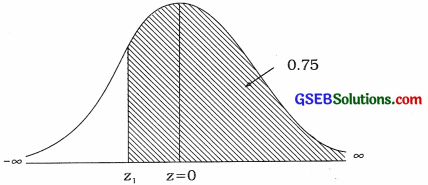P[Z ≥ z1] = 0.75
∴ P [z1 ≤ Z ≤ 0] = P [z1 ≤ Z < ∞] – P [0 ≤ Z < ∞ ]
= 0.75 – 0.50
= 0.25
From the table, for area 0.2486, z1 = – 0.67 and for area 0.2518, z1 = – 0.68
∴ For area 0.25,
z1 = $$\frac{(-0.67)+(-0.68)}{2}$$
= $$\frac{-1.35}{2}$$
= – 0.675Question 9.
If Z is a standard normal variable and z1 represents the Z-score, then estimate the value of z1 so that the following conditions are satisfied:
(i) P[- 2 ≤ Z ≤ z1] = 0.2857
(i) P[- 2 ≤ Z ≤ z1] = 0.2857∴ P[z1 ≤ Z ≤ 0] = 0.75
= p[ – 2 ≤ Z ≤ 0] – p[- 2 ≤ Z ≤ z1]
= 0.4772 – 0.2857
= 0.1915
From the table, for area 0.1915, z1 = – 0.5

(ii) P [z1 ≤ Z ≤ 1.75] = 0.10
P [z1 ≤ Z ≤ 1.75] = 0.10∴ P[0 ≤ Z ≤ z1]
= p[ 0 ≤ Z ≤ 1.75] – p[z1 ≤ Z ≤ 1.75]
= 0.4599 – 0.10
= 0.3599
From the table, for area 0.3599, z1 = 1.08Question 10.
The monthly production of units in a factory is normally distributed with mean µ and standard deviation σ. The Z-scores corresponding to the production of 2400 units and 1800 units are 1 and – 0.5 respectively. Find its mean and standard deviation.
Here, x1 = 2400, x2 = 1800: z1 = 1, z2 = -0.5
Now, Z = $$\frac{x-\mu}{\sigma}$$
∴ 1 = $$\frac{2400-\mu}{\sigma}$$
∴ σ = 2400 – µ ………… (1)
and – 0.5 = $$\frac{1800-\mu}{\sigma}$$
∴ – 0.5σ = 1800 – µ …………… (2)
Subtracting equation (2) from the (1)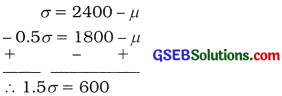∴ σ = $$\frac{600}{1.5}$$ = 400
Putting σ = 400 in equation (1),
400 = 2400 – µ
∴ µ = 2400 – 400 = 2000
Hence, the mean and standard deviation of the distribution obtained are 2000 and 400 respectively.

Question 11.
A normal variable X has the following probability density function:
f(x) = $$\frac{1}{6 \sqrt{2 \pi}} e^{-\frac{1}{72}(x-100)^{2}}$$, – ∞ < x < ∞
For this distribution, obtain the estimated limits for the exact middle 50 % of the observations.
Ans.
f(x) = $$\frac{1}{6 \sqrt{2 \pi}} e^{-\frac{1}{72}(x-100)^{2}}$$, – ∞ < x < ∞
= $$\frac{1}{6 \sqrt{2 \pi}} e^{-\frac{1}{2} \frac{(x-100)^{2}}{6^{2}}}$$, – ∞ < x < ∞
∴ µ = 100, σ = 6
The limits for the exact middle 50 % of the observation is Q1 to Q3.
25 % of observations of distribution are less than Q1 and 25 % observations are more than Q3.
∴ P[X ≤ Q1] = 0.25 and P(X ≥ Q3] = 0.25
z1 = $$\frac{\mathrm{Q}_{1}-\mu}{\sigma}$$ = $$\frac{Q_{1}-100}{6}$$ and z2 = $$\frac{\mathrm{Q}_{3}-\mu}{\sigma}$$ = $$\frac{Q_{3}-100}{6}$$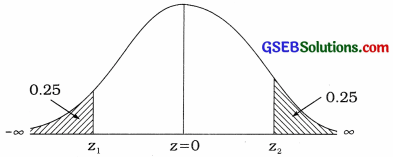∴ P[X ≤ Q1] = P [Z ≤ z1] = 0.25
P [Z ≤ z1] = 0.25
∴ P[z1 ≤ Z ≤ 0] = 0.50 – 0.25 = 0.25
From the table, for area 0.2486, z1 = – 0.67 and for area 0.25 18, z1 = – 0.68
∴ For area 0.25,
z1 = $$\frac{(-0.67)+(-0.68)}{2}$$ = – 0.675
Now, z1 = $$\frac{Q_{1}-100}{6}$$
∴ 0.675 = $$\frac{Q_{1}-100}{6}$$
∴ – 4.05 = Q1 – 100
∴ Q1 = – 4.05 + 100
∴ Q1 = 95.95
Similarly, P [Z ≥ z2] = 0.25
∴ P[0 ≤ Z ≤ z2] = 0.50 – 0.25 = 0.25
As above, for area 0.25, z2 = 0.675
Now, z2 = $$\frac{Q_{3}-100}{6}$$
∴ 0.675 = $$\frac{Q_{3}-100}{6}$$
∴ 4.05 = Q2 – 100
∴ Q2 = 104.05
Hence, the limits for exact middle 50 % of the observations obtained is 95.95 to 104.05.Question 12.
For a normal distribution, the first quartile is 35 and its third quartile is 65. Estimate the limits that includes exactly middle 95.45 % of the observations.
Ans.
Here, Q1 = 35; Q3 = 65
∴ M = $$\frac{Q_{3}+Q_{1}}{2}$$ = $$\frac{65+35}{2}$$ = $$\frac{100}{2}$$ = 50
and quartile deviation = $$\frac{Q_{3}-Q_{1}}{2}$$
= $$\frac{65-35}{2}$$
= $$\frac{30}{2}$$ = 15
Now, in normal distribution µ = M
∴ µ = 50
Quartile deviation = $$\frac{2}{3}$$σ
∴ 15x = $$\frac{2}{3}$$σ
∴ 15 × $$\frac{3}{2}$$ = σ
∴ σ = $$\frac{45}{2}$$ = 22.5
The limits that include exactly middle 95.45 % of the observations = µ ± 2σ
= (µ – 2σ) to (µ + 2σ)
Putting, µ = 50 and σ = 22.5
The limits that include exactly middle 95.45 % of the observations
= [50 – 2(22.5)] to (50 + 2(22.5)]
=(50 – 45) to (50 + 45)
= 5 to 95
Hence, the limits that include exactly middle 95.45 % of the observations for a normal distribution obtained is (5, 95).

Question 13.
For a normal distribution, the third quartile and quartile deviations are 36 and 24 respectively. Find the mean of the distribution.
Ans.
Here, Q3 = 36 and mean deviation = 24.
Now, mean deviation = $$\frac{4}{5}$$ σ
∴24 = $$\frac{4}{5}$$ σ
∴ 24 × $$\frac{5}{4}$$ = σ
∴ σ = 30
Q3 = 36
∴ 25 % of the observations of the distributions are more than 36.
For x = 36, z1 = $$\frac{36-\mu}{\sigma}$$ = $$\frac{36-\mu}{30}$$
Now, P [X ≥ 36] = 0.25
∴ P[Z ≥ z1] = 0.25∴ P[0 ≤ Z ≤ z1] = 0.50 – 0.25 = 0.25
From the table, for area 0.2486, z1 = 0.67 and for area 0.2518, z1 = 0.68
∴ For area 0.25,
z1 = $$\frac{0.67+0.68}{2}$$ = 0.675
Now, z1 = $$\frac{36-\mu}{30}$$
∴ 0.675 = $$\frac{36-\mu}{30}$$
∴ 0.675 × 30 = 36 – µ
∴ 20.25 = 36 – µ
∴ µ = 36 – 20.25
∴ µ = 15.75
Hence, the mean of the distribution obtained is 15.75.Question 14.
A normal variable X has mean 200 and variance 100.
(i) Estimate the values of extreme quartiles.
(ii) Find the approximate value of quartile deviation,
(iii) Find the approximate value of mean deviation.
Here, µ = 200, σ2 = 100, ∴ σ = 10

(i) Extreme quartiles Q1 Q3:
25 % of the observations of the distribution is less than Q1 and 25 % of observations of the distribution Is more than Q3.
For Q1, z1 = $$\frac{Q_{1}-\mu}{\sigma}$$ = $$\frac{Q_{1}-200}{10}$$ and
For Q3, z3 = $$\frac{Q_{3}-\mu}{\sigma}$$ = $$\frac{Q_{3}-200}{10}$$P[X ≤ Q1] = P[Z ≤ z1] = 0.25 and
P[X ≥ Q3] = P[Z ≥ z2] = 0.25
∴ P [z1 ≤ Z ≤ 0] = 0.50 – 0.25 = 0.25 and
P [0 ≤ Z ≤ z2] = 0.50 – 0.25 = 0.25
For area 0.2486, z1 = -0.67 and for area 0.2518, z1 = – 0.68
For area 0.25, z1 = $$\frac{(-0.67)+(-0.68)}{2}$$
= – 0.675
and for area 0.25.
z2 = $$\frac{0.67+0.68}{2}$$ = 0.675
Now, z1 = $$\frac{Q_{1}-200}{10}$$
∴ – 0.675 = $$\frac{Q_{1}-200}{10}$$
∴ – 6.75 = Q1 – 200
∴ Q1 = 200 – 6.75
∴ Q1 = 193.25

Now, z2 = $$\frac{Q_{3}-200}{10}$$
∴ 0.675 = $$\frac{Q_{3}-200}{10}$$
∴ 6.75 = Q3 – 200
∴ Q3 = 200 + 6.75
∴ Q3 = 206.75

(ii) The approximate value of quartile deviation:
In normal distribution, quartile deviation ≈ $$\frac{2}{3}$$σ
Putting, σ = 10
Quartile deviation ≈ $$\frac{2}{3}$$ × 10 = $$\frac{20}{3}$$ ≈ 6.67
Hence, the approximate value of quartile deviation obtained is 6.67.

(iii) Approximate value of mean deviation:
In normal distribution, mean deviation ≈ $$\frac{4}{5}$$σ
Putting, σ = 10
Mean deviation ≈ $$\frac{4}{5}$$ × 10 ≈ 8
Hence, the approximate value of mean deviation obtained is 8.Section E

Solve the following:

Question 1.
An amount of purchase of a customer in a mall of a city follows normal distribution with mean₹ 800 and standard deviation ₹ 200. If a customer is selected at random, then find the probabilities for the following events:
(1) Amount of purchase made by him is in between ₹ 850 to ₹ 1200.
(2) Amount of purchase made by him is in between ₹ 600 to ₹ 750.
Here, µ = 800; σ = 200
X = Amount of purchase (₹)

(1) Amount of purchase is between ₹ 850 to ₹ 1200:
P[850 ≤ X ≤ 1200] = ?
For x1 = 850,
z1 = $$\frac{x_{1}-\mu}{\sigma}$$ = $$\frac{850-800}{200}$$ = $$\frac{50}{200}$$ = 0.25
For x2 = 1200,
z2 = $$\frac{x_{2}-\mu}{\sigma}$$ = $$\frac{1200-800}{200}$$ = $$\frac{400}{200}$$ = 2.00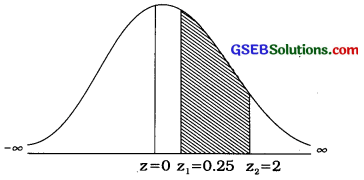P[850 ≤ X ≤ 1200] = P[0.25 ≤ Z ≤ 2]
= P[0 ≤ Z ≤ 2] – P[0 ≤ Z ≤ 0.25]
= 0.4772 – 0.0987
= 0.3785
Hence, the probability that the amount of purchase is between ₹ 850 to ₹ 1200, obtained is 0.3785.

(2) Amount of purchase is between ? 600 and f 750:
P[600 ≤ X ≤ 750] = ?
For x1 = 600,
z1 = $$\frac{x_{1}-\mu}{\sigma}$$ = $$\frac{600-800}{200}$$ = $$\frac{-200}{200}$$ = – 1
For x2 = 750,
z2 = $$\frac{x_{2}-\mu}{\sigma}$$ = $$\frac{750-800}{200}$$ = $$\frac{-50}{200}$$ = – 0.25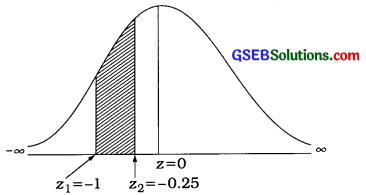P[600 ≤ X ≤ 750] = P[- 1≤ Z ≤ – 0.25]
= P[- 1 ≤ Z ≤ 0] – P[- 0.25 ≤ Z ≤ 0]
= P[0 ≤ Z ≤ 1] – P[0 ≤ Z ≤ 0.25]
= 0.3413 – 0.0987
= 0.2426Question 2.
The average weight of 500 persons of age between 20 years and 26 years of certain area is 55 kg and its variance is 100 (kg)2. The weight of these persons follows normal distribution. According to the weight of persons they can be categorized as under:
(1) Person having weight more than 70 kg is in the fat persons group.
(2) Person having weight between 50 kg to 60 kg is in the healthy persons group.
(3) Person having weight less than 35 kg is in the physically weak person’s group.
From this information, estimate the number of fat persons, number of healthy persons and number of physically weak persons in that area.
Here, N = 500; µ = 55; σ2 = 100 ∴ σ = 10
X = Average weight (in kg)

(1) Persons having weight more than 70 kg is in the fat persons group:
So, P[X ≥ 70] = P[X ≥ x1] = ?
For x1 = 70,
z1 = $$\frac{x_{1}-\mu}{\sigma}$$ = $$\frac{70-55}{10}$$ = $$\frac{15}{10}$$ = 1.5
∴ P[Z ≥ z1] = P[Z ≥ 1.5]P[Z ≥ 1.5] = P[0 ≤ Z < ∞] – P[0 ≤ Z ≤ z1]
= P[0 ≤ Z < ∞] – P[0 ≤ Z ≤ 1.5]
= 0.5000 -0.4332
= 0.0668
Hence, the estimated number of fat persons = N × P[Z ≥ 1.5]
= 500 × 0.0668
= 33.4
≈ 33 persons(2) Persons having weight between 50 kg to 60 kg is in the healthy persons group:
So, P[50 ≤ X ≤ 60] = P[x1 ≤ X ≤ x2] = ?
For x1 = 50.
z1 = $$\frac{x_{1}-\mu}{\sigma}$$ = $$\frac{50-55}{10}$$ = $$\frac{-5}{10}$$ = – 0.5
For x2 = 60.
z2 = $$\frac{x_{2}-\mu}{\sigma}$$ = $$\frac{60-55}{10}$$ = $$\frac{5}{10}$$ = 0.5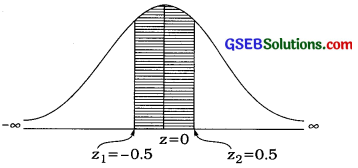∴ P[50 ≤ X ≤ 60] = P[- 0.5 ≤ Z ≤ 0.5]
= P[- 0.5 ≤ Z ≤ 0] + P[0 ≤ Z ≤ 0.5]
= P[0 ≤ Z ≤ 0.5] + P[0 ≤ Z ≤ 0.5]
= 2[0 ≤ Z ≤ 0.5]
= 2[0.1915] = 0.3830
Hence, the estimated number of healthy
persons = N × P [- 0.5 ≤ Z ≤ 0.51
= 500 × 0.3830
= 191.5 ≈ 192 persons

(3) Person having weight less than 35 kg is in the physically weak person’s group.
So, P[X ≤ 35] = P[X ≤ x1] = ?
For x1 = 35,
z1 = $$\frac{x_{1}-\mu}{\sigma}$$ = $$\frac{35-55}{10}$$ = $$\frac{-20}{10}$$ = – 2P[Z ≤ 35] = P[Z ≤ – 2]
= P[- ∞ < Z ≤ 0] – P[- 2 ≤ Z ≤ 0]
= P[0 ≤ Z < ∞] – P[0 ≤ Z ≤ 2]
= 0.5000 -0.4772
= 0.0668
Hence, the estimated number of fat persons
= N × P[Z ≤ – 2]
= 500 × 0.0668
= 33.4
≈ 11 personsQuestion 3.
The average monthly expense of students residing in university hostel is ₹ 2000 and its standard deviation is ₹ 500. If the monthly expense of a student follows normal distribution, then
(1) Find percentage of students having expense between ₹ 750 and ₹ 1250.
(2) Find percentage of students having expense more than ₹ 1800.
(3) Find percentage of students having expense less than ₹ 2400.
Here, µ = 2000; σ = 500; X = Average monthly expense (in ₹).

(1) The percentage of students having expense between ₹ 750 and ₹ 1250 :
P[750 ≤ X ≤ 1250] = ?
For x1 = 750,
z1 = $$\frac{x_{1}-\mu}{\sigma}$$ = $$\frac{750-2000}{500}$$ = $$\frac{-1250}{500}$$ = – 2.5
For x2 = 1250,
z2 = $$\frac{x_{2}-\mu}{\sigma}$$ = $$\frac{1250-2000}{500}$$ = $$\frac{-750}{500}$$ = – 1.5∴ P[750 ≤ X ≤ 1250]
= P[- 2.5 ≤ Z ≤ – 1.5]
= P[- 2.5 ≤ Z ≤ 0] – P[- 1.5 ≤ Z ≤ 0]
= P[0 ≤ Z ≤ 2.5] – P[0 ≤ Z ≤ 1.5]
= 0.4938 – 0.4332
= 0.0606
Hence, the percentage of students having expense between ₹ 750 and ₹ 1250 = 0.0606 × 100 = 6.06%.

(2) The percentage of students having expense more than ₹ 1800:
P[X ≥ 1800] = ?
For x1 = 1800,
z1 = $$\frac{x_{1}-\mu}{\sigma}$$ = $$\frac{1800-2000}{500}$$ = $$\frac{-200}{500}$$ = – 0.4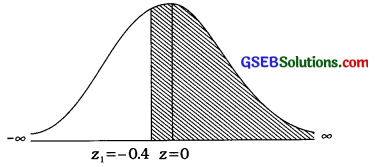∴ P[X ≥ 1800] = P[Z ≥ – 0.4]
= P[- 0.4 ≤ Z ≤ 0] + P[0 ≤ Z < ∞]
= P[0 ≤ Z ≤ 0.4] + P[0 ≤ Z < ∞]
= 0.1554 + 0.5000
= 0.6554
Hence, the percentage of students having expense more than ₹ 1800 = 0.6554 × 100 = 65.54%.(3) The percentage of students having expense less than ₹ 2400:
P[X ≤ 2400] = ?
For x1 = 2400,
z1 = $$\frac{x_{1}-\mu}{\sigma}$$ = $$\frac{2400-2000}{500}$$ = $$\frac{400}{500}$$ = 0.8∴ P[X ≤ 2400] = P[Z ≤ 0.8]
= P[- ∞ < Z ≤ 0] + P[0 ≤ Z =≤ 0.8]
= P[0 ≤ Z < ∞] + P[0 ≤ Z ≤ 0.8]
= 0.5000 + 0.2881
= 0.7881
Hence, the percentage of students having expense less than ₹ 2400
= 0.7881 × 100 = 78.81%.

Question 4.
The monthly average salary of workers working in a production house is ₹ 10000 and its standard deviation is ₹ 2000. By assuming that the monthly salary of a worker follows normal distribution, estimate the maximum salary of 20% of the workers having lowest salary. Also estimate the minimum salary of 10 % of the workers having highest salary.
Here, X = Monthly salary; µ = 10000; σ = 2000

Maximum salary of 20 % workers having lowest salary:
Suppose, maximum salary = ₹ x1
∴ P[X ≤ x1] = $$\frac{20}{100}$$ = 0.2
For x1, z1 = $$\frac{x_{1}-\mu}{\sigma}$$ = $$\frac{x_{1}-10000}{2000}$$
∴ P[Z ≤ z1] = 0.2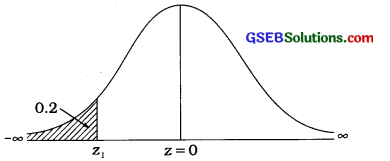P[Z ≤ z1] = 0.2
= P[- ∞ < Z ≤ 0] – P[z1 ≤ Z ≤ 0]
= P[0 ≤ Z < ∞] – P[0 ≤ Z ≤ z1]
∴ P[0 ≤ Z ≤ z1] = 0.5000 – 0.2000
= 0.3000
From the table for area 0.2995, z1 = – 0.84 and for area 0.3023,
z1 = – 0.85 and for area $$\frac{0.2995+0.3023}{2}$$ = 0.3009,
z1 = $$\frac{(-0.84)+(-0.85)}{2}$$ = – 0.845
(∵ z1 is on left side.)
According to the above calculation, 0.3000 is very near to 0.2995, so we will take z1 = – 0.84.
Now, z1 = $$\frac{x_{1}-10000}{2000}$$
∴ – 0.84 = $$\frac{x_{1}-10000}{2000}$$
∴ – 0.84 × 2000 = x1 – 10000
∴ – 1680 = x1 – 10000
∴ 10000 – 1680 = x1
∴ x1 = 8320
Hence, the maximum salary of 20 % of workers having lowest salary is ₹ 8320.

The minimum salary of 10 % of workers having maximum salary:
Suppose, maximum salary = ₹ x2
∴ P[X ≥ x1] = $$\frac{10}{100}$$ = 0.1
For x2, z2 = $$\frac{x_{2}-\mu}{\sigma}$$ = $$\frac{x_{2}-10000}{2000}$$
∴ P[Z ≥ z2] = 0.1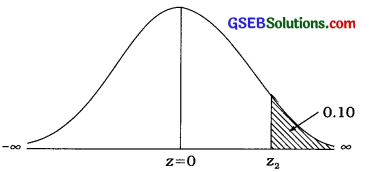P[Z ≥ z2] = 0.2
= P[0 ≤ Z < ∞] – P[0 ≤ Z ≤ z2]
∴ P[0 ≤ Z ≤ z2] = = P[0 ≤ Z < ∞] – P[Z ≥ z2]
= 0.5000 – 0.1000
= 0.4000
From the table for area 0.2995, z2 = 1.28 and for area 0.4015,
z2 = 1.29 and for area $$\frac{0.3997+0.4015}{2}$$ = 0.4006,
z2 = $$\frac{1.28+1.29}{2}$$ = – 1.285
According to the above calculation, 0.4000 is near to 0.3997, so we will take z2 = 1.28.
Now, z2 = $$\frac{x_{2}-10000}{2000}$$
∴ 1.28 = $$\frac{x_{1}-10000}{2000}$$
∴ 1.28 × 2000 = x2 – 10000
∴2560 = x2 – 10000
∴ x2 = 10000 + 2560
∴ x2 = 12560
Hence, the maximum salary of 10 % of workers having maximum salary is ₹ 12560.Question 5.
A normal distribution has mean 52 and variance 64. Obtain estimated limits which include exactly middle 25 % of the observations.
Here, µ = 52; σ2 = 64 ∴ σ = 8 .
Suppose, the limits that include exactly middle 25% of the observations is x1 to x2.
∴ P[x1 ≤ X ≤ x2] = $$\frac{25}{100}$$ = 0.25
∴ Px1 ≤ X ≤ µ] = P[µ ≤ X ≤ x2] = $$\frac{0.25}{2}$$ = 0.125
For x1, z1 = $$\frac{x_{1}-\mu}{\sigma}$$ = $$\frac{x_{1}-52}{8}$$ and
For x2, z2 = $$\frac{x_{2}-\mu}{\sigma}$$ = $$\frac{x_{2}-52}{8}$$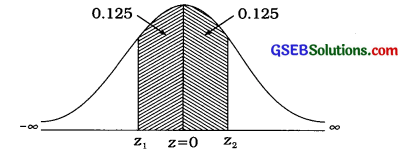P[x1 ≤ X ≤ µ] = 0.125
∴ P[z1 ≤ Z ≤ 0] = 0.125
From the table, for area 0.1217, z1 = – 0.31 and for area 0.1255,
z1 = – 0.32 and for area $$\frac{0.1217+0.1255}{2}$$ = 0.1236,
z1 = $$\frac{(-0.31)+(-0.32)}{2}$$ = – 0.315
(∵ z1 is on left side.)
According to above calculation, 0.125 is near to 0.1255, so we will take z1 = -0.32.
Now, z1 = $$\frac{x_{1}-52}{8}$$
∴ – 0.32 = $$\frac{x_{1}-52}{8}$$
∴ – 0.32 × 8 = x1 – 52
∴ 52 – 2.56 = x1
∴ x1 = 49.44
P[µ ≤ X ≤ x2] = 0.125
∴ P[0 ≤ Z ≤ z2] = 0.125 (∵ z2 = 0.32 ( vz2 is on right side.)
Now, z2 = $$\frac{x_{2}-\mu}{\sigma}$$
∴ 0.32 = $$\frac{x_{2}-52}{8}$$
∴ 0.32 × 8 = x2 – 52
∴ 52 + 2.56 = x2
∴ x2 = 54.56
Hence, the limits which include exactly middle 25% of the observations of a normal distribution obtained is 49.44 to 54.56.

Question 6.
In a big showroom of electronic items, on an average 52 electronic units are sold every week and its variance is 9 (unit)2. Sale of electronic items follows normal distribution. The probability that the sale of electronic items during a week out of 50 weeks is from x1 units to 61 units is 0.1574. Estimate the value of x1. Also estimate the number of weeks during which the sale of electronic items is more than 55 units.
Here, µ = 52; σ2 = 9 ∴ σ = 3; N = 50
Probability that the sale of electronic item is from xx units to 61 items is 0.1574.
∴ P[x1 ≤ X ≤ 61] = 0.1574
For x1, z1 = $$\frac{x_{1}-\mu}{\sigma}$$ = $$\frac{x_{1}-52}{3}$$
For x1, z1 = $$\frac{x_{2}-\mu}{\sigma}$$ = $$\frac{61-52}{3}$$ = $$\frac{9}{3}$$ = 3
∴ P[z1 ≤ Z ≤ z2] = 0.1574Now, P[0 ≤ Z ≤ z2] = P[0 ≤ Z ≤ 3] = 0.4987
∴ P[0 ≤ Z ≤ z1] = P[0 ≤ Z ≤ 3] – P[z1 ≤ Z ≤ z2]
= 0.4987 – 0.1574
= 0.3413
For area 0.3413, z1 = 1
Now, z1 = $$\frac{x_{1}-\mu}{\sigma}$$
∴ 1 = $$\frac{x_{1}-52}{3}$$
∴ 1 × 3 = x1 – 52
∴ 3 + 52 = x1
∴ x1 = 55 units
The sale of electronic items is more than 55 units:
P[X ≥ 55] = ?
For x1 = 55, z1 = $$\frac{x_{1}-\mu}{\sigma}$$ = $$\frac{55-52}{3}$$ = $$\frac{3}{3}$$ = 1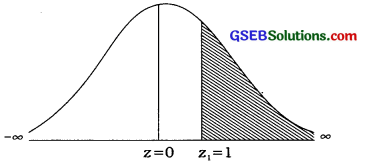P[Z ≥ 55] = P[0 ≤ Z< ∞] – P[0 ≤ Z ≤ 1]
= 0.5000 – 0.3413
= 0.1587
Hence, the number of weeks during which the sale of electronic items is more than 55 units
= N × P[Z ≥ 1]
= 50 × 0.1587
= 7.935 ≈ 8 weeksQuestion 7.
It is known that on an average a person spends 61 minutes in a painting exhibition. If this time is normally distributed and 20 % of the persons spent less than 30 minutes in the exhibition then find variance of the distribution. Also determine the probability that a person spends more than 90 minutes in the exhibition.
Here, µ = 61; P[X ≤ 30] = $$\frac{20}{100}$$ = 0.2 are given.

Variance of distribution:
For x1 = 30, z1 = $$\frac{30-\mu}{\sigma}$$
= $$\frac{30-61}{\sigma}$$ = $$\frac{-31}{\sigma}$$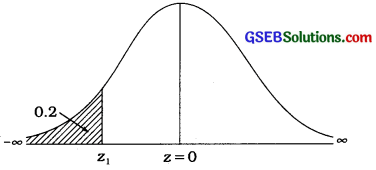∴ P[Z ≤ z1] = 0.2
Now, P[z1 ≤ Z ≤ 0] = P[- ∞ < Z ≤ 0] – P[Z ≤ z1]
= 0.5000 – 0.2000
= 0.3000
For area 0.2995 ≈ 0.3000, z1 = – 0.84
Now, z1 = $$\frac{-31}{\sigma}$$
∴ – 0.84 = $$\frac{-31}{\sigma}$$
∴ σ = $$\frac{-31}{-0.84}$$ = 36.9 (Approximately)
∴ σ2 = 1361.61 (Approximately) is given
In the textbook σ2 = 1362.25

A person spends more than 90 minutes in the exhibition:
P[X ≥ 90]
For x = 90, z2 = $$\frac{x_{2}-\mu}{\sigma}$$ = $$\frac{90-61}{36.9}$$ = 0.7859 ≈ 0.79P[Z ≥ z2] = P[0 ≤ Z < ∞] – P[0 ≤ Z ≤ z2]
= P[0 ≤ Z < ∞] – P[0 ≤ Z ≤ 0.79]
= 0.5000 – 0.2852
= 0.2148
Hence, the probability that a person spends more than 90 minutes in the exhibition obtained is 0.2148.Question 8.
If the diameter of pipes produced by a company manufacturing pipes is 20 mm to 22 mm, then it is accepted by specified group of customers. The standard deviation of produced pipes is 4 mm and it is known that 70 % of the pipes produced in the unit have diameter more than 19.05 mm. Find the average diameter of the produced pipes. Also find the percentage of pipes rejected by specified group of customers. Note: The diameter of the produced pipes follows normal distribution.
Here, σ = 4, P[X ≥ 19.05] = $$\frac{70}{100}$$ = 0.7 are given

Mean of the distribution:
For x1 = 19.05, z1 = $$\frac{x_{1}-\mu}{\sigma}$$ = $$\frac{19.05-\mu}{4}$$∴ P[Z ≥ z1] = P[z1 ≤ Z ≤ 0] + P[0 ≤ Z < ∞]
∴ P[0 ≤ Z ≤ z1] = P[Z ≥ z1] – P [0 ≤ Z < ∞]
= 0.7000 – 0.5000
= 0.2000
For area 0.1985, z1 = – 0.52 and for area 0.2019, z1 = – 0.53
∴ z1 = $$\frac{(-0.52)+(-0.53)}{2}$$ = – 0.525
Now, z1 = $$\frac{x_{1}-\mu}{\sigma}$$
∴ – 0.525 = $$\frac{19.05-\mu}{4}$$
∴ – 0.525 × 4 = 19.05 – µ
∴ – 2.1 = 19.05 – µ
∴ µ = 19.05 + 2.1 = 21.15
Hence, the mean of the diameter of the produced pipes obtained is 21.15 mm.

Probability that the diameter of pipes lie between 20 mm and 22 mm:
P[20 ≤ X ≤ 22]
For x1 = 20,
z1 = $$\frac{x_{1}-\mu}{\sigma}$$ = $$\frac{20-21.15}{4}$$ = – 0.2875 ≈ – 0.29
For x2 = 22,
z2 = $$\frac{x_{2}-\mu}{\sigma}$$ = $$\frac{22-21.15}{4}$$ = – 0.2125 ≈ – 0.21∴ P[20 ≤ X ≤ 22]
= P[- 0.29 ≤ Z ≤ 0.21]
= P[- 0.29 ≤ Z ≤ 0] + P[0 ≤ Z ≤ 0.21]
= P[0 ≤ Z ≤ 0.29] + P[0 ≤ Z ≤ 0.21]
= 0.1141 + 0.0832
= 0.1973
Hence, the probability that the pipes are accepted by the customer obtained is 0.1973.
∴ Probability that pipes are rejected = 1 – 0.1973
= 0.8027
Hence, the percentage of rejected pipes by customer = 0.8027 × 100 = 80.27%Question 9.
A normal variable X has mean 400 and variance 900. FInd the fourth decile and 90th percentile for this distribution and also interpret the values.
Here, µ = 400; σ2 = 900 ∴ σ = 30
Fourth Decile D4:
40% of the observations are less than D4.
∴ P[x ≤ D4] = $$\frac{40}{100}$$ = 0.40
For D4 , z1 = $$\frac{\mathrm{D}_{4}-\mu}{\sigma}$$ = $$\frac{\mathrm{D}_{4}-400}{30}$$P[X ≤ D4] = P[Z ≤ Z1] = 0.40
∴ P[z1 ≤ Z ≤ 0] = P[- ∞ < Z ≤ 0] – P[Z ≤ z1]
= 0.50 – 0.40
= 0.10
For area 0.0987, z1 = – $$\frac{(-0.25)+(-0.26)}{2}$$ = $$\frac{-0.51}{2}$$ = – 0.255
Now, z1 = $$\frac{\mathrm{D}_{4}-400}{30}$$
∴ – 0.255 = $$\frac{\mathrm{D}_{4}-400}{30}$$
∴ – 0.255 × 30 = D4 – 400
∴ – 7.65 = D4 – 400
∴ D4 = 400 – 7.65 = 392.35
Hence, the fourth decile D4 obtained is 392.35.

Interpretation: 40 % of the observations of the given distribution are less than 392.35.

90th Percentile P9o:
90% of the observations are less than P9o.
∴ p[X ≤ P9o] = $$\frac{90}{100}$$ = 0.90
For P90, z2 = $$\frac{\mathrm{P}_{90}-\mu}{\sigma}$$ = $$\frac{\mathrm{P}_{90}-400}{30}$$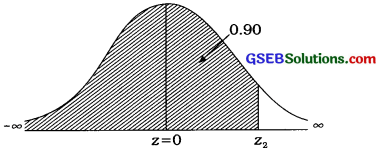P[X ≤ P9o] = P[Z ≤ Z2] = 0.90
∴ P[0 ≤ Z ≤ z2] = P[Z ≤ z2] – P[- ∞ < Z ≤ 0]
= 0.90 – 0.50
= 0.40
For area 0.3997 ≈ 0.40, z2 = 1.28
Now, z2 = $$\frac{\mathrm{P}_{90}-400}{30}$$
∴ 1.28 = $$\frac{\mathrm{P}_{90}-400}{30}$$
∴ 1.28 × 30 = P9o – 400
∴ 38.4 = P9o – 400
∴ P9o = 38.4 + 400
∴ P9o = 438.4
Hence, the 90th percentile P9o obtained is 438.4.
Interpretation: 90 % of the observations of the given distribution are less than 438.4.Question 10.
A normal variable X has the following density function:
f(x) = $$\frac{1}{50 \sqrt{2 \pi}} e^{-\frac{1}{2}\left(\frac{x-150}{50}\right)^{2}}$$, – ∞ < x < ∞
For this distribution,
(1) If P[x1 ≤ X ≤ 250] = 0.4772, then estimate x1
(2) If P[75 ≤ X ≤ x2] = 0.3539, then estimate x2.
Here, f(x) = $$\frac{1}{50 \sqrt{2 \pi}} e^{-\frac{1}{2}\left(\frac{x-150}{50}\right)^{2}}$$, – ∞ < x < ∞
∴ µ = 150, σ = 50

(1) If P[x1 ≤ X ≤ 250] = 0.4772,
For 250, z2 = $$\frac{250-150}{50}$$ = $$\frac{100}{50}$$ = 2
For x1, z1 = 0 (∵ P(0 ≤ Z ≤ 2] = 4.4772)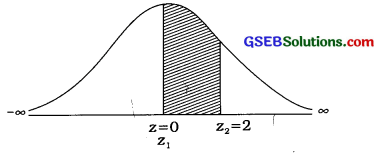P[x1 ≤ X ≤ 250] = 0.4772
∴ P[0 ≤ Z ≤ 2] = 0.4772
Now, z1 = $$\frac{x_{1}-150}{50}$$
∴ 0 = $$\frac{x_{1}-150}{50}$$
∴ 0 = x1 – 150
∴ x1 = 150

(2) If P[75 ≤ X ≤ x2] = 0.3539,z1 = $$\frac{75-\mu}{\sigma}$$ = $$\frac{75-150}{50}$$ = – $$\frac{75}{50}$$ = – 1.5
z2 = $$\frac{x_{2}-\mu}{\sigma}$$ = $$\frac{x_{2}-150}{50}$$
P[75 ≤ X ≤ x2] = 0.3539
∴ P[- 1.5 ≤ Z ≤ z2) = 0.3539
∴ P[z2 ≤ Z ≤ 0]
= P[- 1.5 ≤ Z ≤ 0] – P[- 1.5 ≤ Z ≤ z2]
= 0.4332 – 0.3539
= 0.0793
Forn the table, for area 0.0793, z2 = – 0.2
Now, z2 = $$\frac{x_{2}-150}{50}$$
∴ – 0.2 = $$\frac{x_{2}-150}{50}$$
∴- 0.2 × 50 = x2 – 150
∴ – 10 = x2 – 150
∴ x2 = – 10 + 150 = 140
Hence, the estimated value of x2 obtained is 140.Section F

Solve the following:

Question 1.
An Intelligence test is conducted for 500 children and it is found that the average marks are 68 and standard deviations is 22. If the marks obtained by the children is normally distributed, then
(1) Find the number of children getting marks more than 68.
(2) Find the percentage of children getting marks between 70 and 90.
(3) Find the minimum score of most intelligent 50 children.
Here, N = 500; µ = 68; σ = 22

(1) Number of children getting marks more than 68:
For x = 68, z1 = $$\frac{x_{1}-\mu}{\sigma}$$ = $$\frac{68-68}{22}$$ = 0
P[X ≥ 68] = P[Z ≥ z1] = P[Z ≥ 0]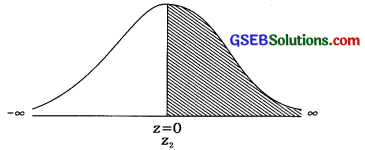P[Z ≥ 0] = 0.5
Hence, the number of children getting marks more than 68
= N × P[Z ≥ 0]
= N × P[Z ≥ 0]
= 500 × 0.5
= 250

(2) Find the percentage of children getting marks between 70 and 90.
For x1 = 70, z1 = $$\frac{70-68}{22}$$ = $$\frac{2}{22}$$ = $$\frac{1}{11}$$ = 0.09
For x2 = 90, z2 = $$\frac{90-68}{22}$$ = $$\frac{22}{22}$$ = 1Now, P[70 ≤ Z ≤ 90]
= P[0.091 ≤ Z ≤ 1]
= P[0 ≤ Z ≤ 1] – p[0 ≤ Z ≤ 0.09]
= 0.3413 – 0.0359
= 0.3054
Hence, the percentage of children getting marks between 70 and 90
= 0.3054 × 100
= 30.54%

(3) Minimum score of most IntellIgent 50 children:
Suppose, minimum score of 50 chIldren is = x1
∴ P(X ≥ x1] = $$\frac{50}{500}$$ = 0.1
For x1, z1 = $$\frac{x_{1}-68}{22}$$
∴ P(Z ≥ z1] = 0.1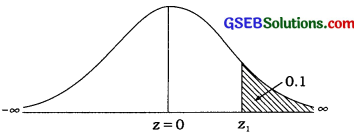Now,
P[0 ≤ Z ≤ z1] = P[0 ≤ Z < ∞] – P[Z ≥ z1]
= 0.5 – 0.1
= 0.4
For area 0.3997 ≈ 0.4, z1=1.28
Now, z1 = $$\frac{x_{1}-68}{22}$$
∴ 1.28 = $$\frac{x_{1}-68}{22}$$
∴ 1.28 × 22 = x1 – 68
∴ 28.16 = x1 – 68
∴ x1 = 28.16 + 68
∴ x1 = 96.16
≈ 96 marks
Hence, the minimum score of most intelligent 50 children is approximately 96 marks.Question 2.
Age of 500 employees working in a private company follows normal distribution with mean 40 years and standard deviation 6 years. The company wants to reduce its staff by 25 % in the following manner:
(1) To retrench 5 % of the employees having minimum age.
(2) After retrenching 5 % of employees having minimum age, next 10 % of the employees are to be transferred to another company.
(3) To retire 10 % of employees having maximum age.
From this information, find the age of employees who are to be retrenched, transferred and retired from the company.
Here, X = Age; µ = 40; σ = 6

(1) To retrench 5 % of the employees having minimum age.
Suppose, this age is x1.
∴ P[X ≤ x1] = $$\frac{5}{100}$$ = 0.05
For x1, z1 = $$\frac{x_{1}-40}{6}$$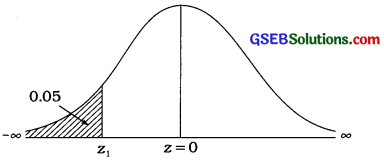P[Z ≤ Z1] = 0.05
∴ P[0 ≤ Z ≤ z1] = P[0 ≤ Z < ∞] – P[Z ≤ z1]
= 0.5000 – 0.05
= 0.4500
For area 0.4495, z1 = – 1.64 and for area 0.4505, z1 = – 1.65
∴ For area 0.45,
z1 = $$\frac{(-1.64)+(-1.65)}{2}$$
Now, z1 = $$\frac{x_{1}-40}{6}$$
∴ – 1.645 = $$\frac{x_{1}-40}{6}$$
∴ – 1.645 × 6 = x1 – 40
∴ – 9.87 = x1 – 40
∴ 40 – 9.87 = x1 = 30.13
∴ x1 = 30.13 years
Hence, the minimum age of employees retrenched obtained is 30.13 years.

(2) After retrenchment next 10 % of the employees to be transferred to another company:
5 % of the employees having minimum age, is retrenched and next 10 % of the employees are transferred to another company. Thus, total percentage of employees comes to 15 %.
∴ P[X ≤ x2] = $$\frac{15}{100}$$ = 0.05
For x2, z2 = $$\frac{x_{2}-40}{6}$$P[Z ≤ Z2] = 0.15
∴ P[0 ≤ Z ≤ z2] = P[-∞ < Z ≤ 0] – P[Z ≤ z2]
= 0.5000 – 0.05
= 0.3500
For area 0.3485, z2 = – 1.03 and for area 0.3508, z2 = – 1.04
∴ For area 0.35,
z2 = $$\frac{(-1.03)+(-1.04)}{2}$$
Now, z2 = $$\frac{x_{2}-40}{6}$$
∴ – 1.035 = $$\frac{x_{2}-40}{6}$$
∴ – 1.035 × 6 = x2 – 40
∴ – 6.21 = x2 – 40
∴ 40 – 6.21 = x2 = 33.79
∴ x2 = 30.13 years
Hence, the age of the employees taking transfer to another companies obtained is 33.79 years.

(3) 10% of the employees having maximum age are retired:
∴ P[X ≥ x1] = $$\frac{10}{100}$$ = 0.1
For x1, z1 = $$\frac{x_{2}-40}{6}$$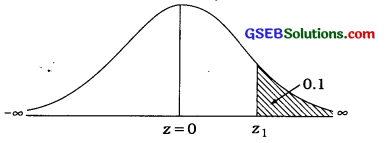P[Z ≥ Z1] = 0.1
∴ P[0 ≤ Z ≤ z1] = P[0 ≤ Z < ∞] – P[Z ≤ z1]
= 0.5000 – 0.1000
= 0.4000
For area 0.3997 ≈ 40000, z1 = 1.28
Now, z1 = $$\frac{x_{1}-40}{6}$$
∴ 1.28 = $$\frac{x_{1}-40}{6}$$
∴1.28 × 6 = x1 – 40
∴7.68 = x1 – 40
∴ 7.68 + 40 = x1
∴ x1 = 47.68 years
Hence, the maximum age of 10% of employees who retire is obtained 47.68 years.Question 3.
An entrance test of 200 marks is conducted for higher studies. 20,000 students remain present in the examination and the marks obtained by them follows normal distribution with mean 120 and standard deviation 20.
The rules for the result are as under:
(1) Students who acquire less than 40 per cent marks are failed.
(2) An additional test is conducted for the students acquiring marks between 40 per cent and 48 per cent.
(3) Students who acquire marks between 48 per cent and 75 per cent are called for personal interview.
(4) Students who acquire marks more than 75 per cent get direct admission for the higher studies.

Find approximate number of students who:
(i) failed In test,
(ii) appeared for additional 100 marks test,
(iii) appeared for personal interview and
(iv) got direct admission for the higher studies.
Here, Z = 20000: µ = 120; σ= 20;
Total marks = 200: X = Marks

(1) Students acqire less than 40 per cent of marks are failed:
40 per cent of 200 marks = $$\frac{200 \times 40}{100}$$ = 80 marks
∴ students acqire less than 80 marks are failed.
∴ P[X ≤ 80] = ?
For x1 = 80,
z1 = $$\frac{x_{1}-\mu}{\sigma}$$ = $$\frac{80-120}{20}$$ = $$\frac{-40}{20}$$ = – 2
∴ P[X ≤ 80] = P[Z ≤ – 2]P[Z ≤ – 2] = P[- ∞ < Z ≤ 0] – P[- 2 ≤ Z ≤ 0]
= P[0 ≤ Z < ∞] – P[0 ≤ Z ≤ – 2]
= 0.5000 – 0.4772
= 0.0228
Hence, the approximate number of students who fail N P[Z ≤ – 2]
= 20000 × 0.0228
= 456 students

(2) An additional test is conducted for the students acquiring marks between 40 per cent and 48 per cent:
40 per cent of 200 marks is 80 marks and 48 per cent of 200 marks is
= $$\frac{200 \times 48}{100}$$ = 96 marks
So, an additional test of 100 marks is conducted for the students acquiring marks between 80 and 96.
P[80 ≤ X ≤ 96] = ?
For x1 = 80,
Z1 = $$\frac{x_{1}-\mu}{\sigma}$$ = $$\frac{80-120}{20}$$ = $$\frac{-40}{20}$$ = – 2
For x2 = 96,
Z2 = $$\frac{x_{2}-\mu}{\sigma}$$ = $$\frac{96-120}{20}$$ = – $$\frac{24}{20}$$ = – 1.2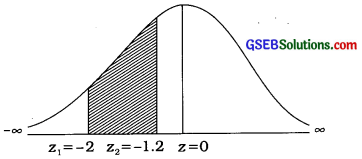∴ P[z1 ≤ Z ≤ z2] = P[- 2 ≤ Z ≤ – 1.2]
= P[- 2 ≤ Z ≤ 0] – P[- 1.2 ≤ Z ≤ 0]
= P[0 ≤ Z ≤ 2] – P[0 ≤ Z ≤ 1.2]
= 0.4772 – 0.3849
= 0.0923
Hence, the approximate number of students who appeared for the additional test of 100 marks = N∙P[- 2 ≤ Z ≤ – 1.2]
= 20000 × 0.0923
= 1846 students

(3) Students who acqire marks between 48 per cent to 75 per cent are called for personal interview:
48 per cent of 200 marks is 96 and 75 per cent of 200 marks is 150 marks.
So, the students who acquire marks between 96 marks and 150 marks are called for personal interview.
P[96 ≤ X ≤ 150] = ?
z1 = – 1.2 and z2 = $$\frac{x_{2}-\mu}{\sigma}$$ = $$\frac{150-120}{20}$$ = 1.5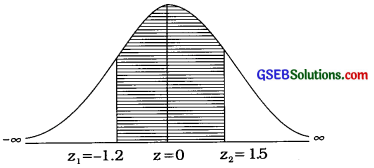P[96 ≤ X ≤ 150] = P[- 1.2 ≤ Z ≤ 1.5]
= P[- 1.2 ≤ Z ≤ 0] + P[0 ≤ Z ≤ 1.5]
= P[0 ≤ Z ≤ 1.2] + P[0 ≤ Z ≤ 1.5]
= 0.3849 + 0.4332 = 0.8181
Hence, the approximate number of students who are called for personal interview
= N∙P[- 1.2 ≤ Z ≤ 1.5]
= 20000 × 0.8181
= 16362 students

(4) Students acquire more than 75 per cent of marks are directly admitted for higher studies:
75 per cent marks of 200 marks =150 marks
∴ P[X ≥ 150] = ?
Z1 = 1.5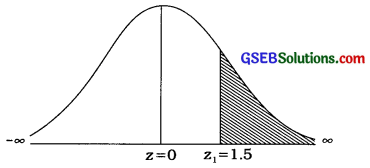∴ P[X ≥ 150] = P[Z ≥ 1.5]
= P[0 ≤ Z < ∞] – P[0 ≤ Z ≤ 1.5]
= 0.5000 – 0.4332
= 0.0668
Hence, the approximate number of students who are directly admitted for higher studies
= N∙P[Z ≥ 1.5]
= 20000 × 0.0668
= 1336 studentsQuestion 4.
The monthly income of a group of persons follows normal distribution with mean ₹ 20000 and standard deviation ₹ 5000. If the minimum monthly income of 50 richest person is ₹ 31625, then how many persons are in the group ? Also, what is the maximum income of 50 persons having lowest monthly income ?
Hence, µ = 20000; σ = 5000;
X = Monthly income
The minimum monthly income of 50 persons having maximum income is ₹ 31625.
∴ P[X ≥ 31625] = ?
For x2 = 31625, z2 = $$\frac{31625-20000}{5000}$$ = $$\frac{11625}{5000}$$ = 2.325 ≈ 2.33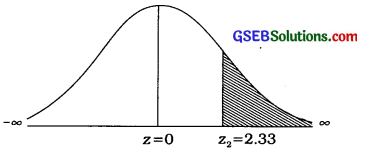Now,
P[Z2 ≥ 2.33] = P[0 ≤ Z < ∞] – P[0 ≤ Z ≤ 2.33]
= 0.5000 – 0.4901
= 0.0099
∴ For area = 0.0099, the number of persons = 50
For area = 1.00, the number of persons
= $$\frac{50}{0.0099}$$
≈ 5051
Hence, the total number of persons in a group will be 5051.
Suppose, the maximum income of 50 persons = ₹ x1
∴ P[X ≤ x1] = $$\frac{50}{5051}$$ ≈ 0.01
For x1, z1 = $$\frac{x_{1}-20000}{5000}$$Now, P[Z ≤ z1] = 0.01
∴ P[z1 ≤ Z ≤ 0] = P[- ∞ < Z ≤ 0] – P[Z ≤ z1]
= 0.5000 – 0.01
= 0.4900
For area 0.4901 ≈ 0.49, z1 = – 2.33
Now, z1 = $$\frac{x_{1}-20000}{5000}$$
∴ – 2.33 = $$\frac{x_{1}-20000}{5000}$$
∴ – 2.33 × 5000 = x1 – 20000
∴ – 11650 = x1 – 20000
∴ x1 = 20000 – 11650
∴ x1 = 8350
Hence, the maximum income of 50 persons having lowest income obtained is ₹ 8350.

Question 5.
Analysis of result of 12th standard students of a school is as under:
Pass with distinction: 15 % of total students
Pass without distinction: 75 % of total students
Fail: 10 % of total students
For passing the examination, minimum 40 % of the total marks and for distinction minimum 80% marks are required. If the percentage of result of the students follows normal distribution then find mean and standard deviation and by using it determine the percentage marks for which 75 % of the students have less than that percentage marks.
Here, X = Percentage of marks obtained
Let, µ = Mean of normal distribution
σ = Standard deviation of normal distribution
Suppose, an examination is conducted of 100 marks. For passing minimum 40 % of the total marks, i.e., 40 marks is required. So the students getting less than 40 marks fails. Now, 10 % of total students fail.
∴ P[X ≤ 40] = $$\frac{10}{100}$$ = 0.1
For x1 = 40, z1 = $$\frac{x_{1}-\mu}{\sigma}$$ = $$\frac{40-\mu}{\sigma}$$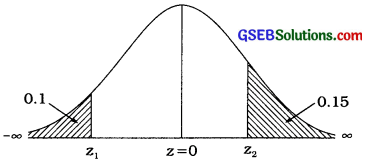Now, P [Z ≤ z1] = 0.1
∴ P[z1 ≤ Z ≤ 0] = P[- ∞ < z ≤ 0] – P[Z ≤ z1]
= 0.5000 – 0.1000
= 0.4000
For area 0.3997 ≈ 0.4000, z1 = – 1.28
z1 = $$\frac{x_{1}-\mu}{\sigma}$$
∴ – 1.28 = $$\frac{40-\mu}{\sigma}$$
∴ – 1.28 σ = 40 – µ …………. (1)
Now, for distinction 80% of marks, i.e., 80 marks are required. So, the students getting more than 80 marks pass with distinction. Now, the percentage of students who pass with distinction is 15 % of total students.
∴ P[x > 80] = $$\frac{15}{100}$$ = 0.15
For x2 = 80, z2 = $$\frac{x_{2}-\mu}{\sigma}$$ = $$\frac{80-\mu}{\sigma}$$
Now, P[Z ≥ z2] = 0.15
∴ P [0 ≤ Z ≤ Z2] = P [0 ≤ Z < ∞] – P [Z ≥ Z2]
= 0.5000 – 0.1500
= 0.3500
For area 0.3485, z2 = 1.03 and for area 0.3508, z2 = 1.04
∴ For area 0.35, z2 = $$\frac{1.03+1.04}{2}$$ = 1.035
∴ z2 = 1.035
Now, z2 = $$\frac{80-\mu}{\sigma}$$
∴ 1.035 = $$\frac{80-\mu}{\sigma}$$
∴ 1.035σ = 80 – µ …………… (2)
Subtracting equation (2) from the equation (1),Putting σ = 17.28 in the equation (1),
– 1.28(17.28) = 40 – µ
∴ µ = 40 + 22.12
= 62.12
Hence, the mean marks of the distribution and its standards deviation obtained are 62.12 and 17.28 marks respectively.

Percentage marks for 75 % of the students:
Suppose, the result of 75 % of student is less than x1 percentage.
∴ P[X ≤ x1] = $$\frac{75}{100}$$ = 0.75
For x1, z1 = $$\frac{x_{1}-62.12}{17.28}$$
∴P[Z ≤ z1] = 0.75
∴ P [0 ≤ Z ≤ z1] = P [Z ≤ z1] – P [- ∞ < Z ≤ 0]
= 0.75 – 0.50
= 0.25
For area 0.2486, z1 = 0.67 and for area 0.2518, z1 = 0.68
∴ For area 0.2500, z1 = $$\frac{0.67+0.68}{2}$$ = 0.675Now, z1 = $$\frac{x_{1}-62.12}{17.28}$$
∴ 0.675 = $$\frac{x_{1}-62.12}{17.28}$$
∴ 0.675 × 17.28 = x1 – 62.12
∴ 11.664 + 62.12 = x1
∴ x1 = 73.78
Hence, the result of 75 % of student is less than 73.78 percentage.Question 6.
The monthly bill amount of regular customers of a provision store follows normal distribution. If 7.78 % customers have monthly bill amount less than ₹ 3590 and 94.52% customers have bill amount less than ₹ 5100, then determine the parameters of the normal distribution. Also determine the interval for monthly bill amount of exactly middle 95% customers.
Here, X = Monthly bill amount of customers
The bill of 7.78 % of customers is less than ₹ 3590.
∴ P [X ≤ 3590] = $$\frac{7.78}{100}$$ = 0.0778
Here, x1 = 3590
∴ z1 = $$\frac{x_{1}-\mu}{\sigma}$$ = $$\frac{3590-\mu}{\sigma}$$
The bill of 94.58 % of customers is less than ₹ 5100.
∴P [X ≤ 5100] = $$\frac{94.52}{100}$$ = 0.9452
Here, x2 = 5100
∴ z2 = $$\frac{x_{21}-\mu}{\sigma}$$ = $$\frac{5100-\mu}{\sigma}$$∴ P [Z ≤ z1] = 0.0778 P
∴ [z1 ≤ Z ≤ 0] = P [- ∞ < Z ≤ 0] – P [Z ≤ z1]
= 0.5000 – 0.0778
= 0.4222
For area = 0.4222, z1 = 1.42 = – 1.42
Now, z1 = $$\frac{x_{1}-\mu}{\sigma}$$ = $$\frac{3590-\mu}{\sigma}$$
∴ – 1.42 = $$\frac{3590-\mu}{\sigma}$$
∴ – 1.42 σ = 3590 – µ …………. (1)
P [Z ≤ z2] = 0.9452
∴ P [0 ≤ Z ≤ z2] = P [Z ≤ z2] – P [- ∞ < Z ≤ 0]
= 0.9452 – 0.5000
= 0.4452
For area 0.4452, z2 = 1.60
z1 = $$\frac{x_{2}-\mu}{\sigma}$$ = $$\frac{5100 -\mu}{\sigma}$$
∴ 1.60 = $$\frac{5100-\mu}{\sigma}$$
∴ 1.60 σ = 5100 – µ ………………. (2)
Subtracting equation (2) from equation (1),Putting σ = 500 in equation (2),
1.60 × 500 = 5100 – µ
800 = 5100 – µ
∴ µ = 5100 – 800
∴ µ = 4300
Hence, the mean and standard deviation of normal distribution obtained are ₹ 4300 and ₹ 500 respectively.

Interval for monthly bill amount of exactly middle 95 % customers :
µ = 4300, σ = 500
Interval for the monthly bill amount of exactly middle 95 % customers
= µ ± 1.96 σ
= (µ – 1.96 σ) to (µ + 1.96σ)
= (4300 – 1.96 × 500) to (4300 + 1.96 × 500)
= (4300 – 980) to (4300 + 980)
= (3320, 5280)
[* In textbook instead of parameter σ, the value of variance σ2 is given.]Question 7.
A normal variable X has following probability density function:
f (x) = $$\frac{1}{\sqrt{5000 \pi}} e^{-\frac{1}{5000}(x-75)^{2}}$$, – ∞ ≤ x ≤ ∞
From this, answer the following questions:
(1) If P [60 ≤ X ≤ x2] = 0.5670, then find x2.
(2) If P [x1 ≤ X ≤ 125] = 0.3979, then find x1
(3) Find P[|X – 50| ≤ 10].
Here,
f (x) = $$\frac{1}{\sqrt{5000 \pi}} e^{-\frac{1}{5000}(x-75)^{2}}$$, – ∞ < x < ∞
= $$\frac{1}{50 \sqrt{2 \pi}} e^{-\frac{1}{2}\left(\frac{x-75}{50}\right)^{2}}$$, – ∞ < x < ∞
∴ µ = 75, σ = 50

(1) If P [60 ≤ X ≤ x2] = 0.5670
z1 = $$\frac{60-\mu}{\sigma}$$ = $$\frac{60-75}{50}$$ = $$\frac{-15}{50}$$ = – 0.3
z2 = $$\frac{x_{2}-\mu}{\sigma}$$ = $$\frac{x_{2}-75}{50}$$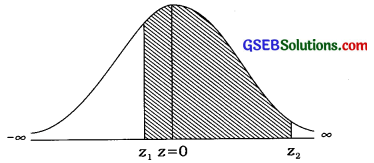P[60 ≤ X ≤ x2] = 0.5670
∴ P [- 0.3 ≤ Z ≤ z2] = 0.5670
∴ P [0 ≤ Z ≤ z2] = P [- 0.3 ≤ Z ≤ z2] – P[- 0.3 ≤ Z ≤ 0]
= 0.5670 – 0.1179
= 0.4491
∴ For area 0.4484, z2 = 1.63 and for area 0.4495, z2 = 1.64
∴ For area 0.4491 z2 = $$\frac{1.63+1.64}{2}$$ = 1.635
Now, z2 = $$\frac{x_{2}-75}{50}$$
∴ 1.635 = $$\frac{x_{2}-75}{50}$$
∴ 50 × 1.635 = x2 – 75
∴ 81.75 = x2 – 75
∴ x2 = 81.75 + 75
∴ x2 = 156.75
Hence, x2 obtained is ≈ 157.

(2) If P [x1 ≤ X ≤ 125] = 0.3979,
z1 = $$\frac{x_{1}-\mu}{\sigma}$$ = $$\frac{x_{1}-75}{50}$$
z2 = $$\frac{125-\mu}{\sigma}$$ = $$\frac{125-75}{50}$$ = $$\frac{50}{50}$$ = – 0.3P[x1 ≤ X ≤ 125] = 0.3979
∴ P[z1 ≤ Z ≤ 1] = 0.3979
∴ P[z1 ≤ Z ≤ 0] = P[z1 ≤ Z ≤ 1] – p[o ≤ Z ≤ 1]
= 0.3979 – 0.3413
= 0.0566
For area 0.0557, z1 = – 0.14
and for area 0.0596. z1 = – 0.15
∴ For area 0.0566 z1 = $$\frac{(-0.14)+(-0.15)}{2}$$ = – 0.145
Now, z1 = $$\frac{x_{1}-75}{50}$$
– 0145 = $$\frac{x_{1}-75}{50}$$
∴ – 0.145 × 50 = x1 – 75
∴ – 7.25 = x1 – 75
∴ x1 = 75 – 7.25 = 67.75 ≈ 68
Hence, x1 obtained is ≈ 68.

(3) Find P[|X – 50| ≤ 10]
P[|X – 50| ≤ 10] = P[40 ≤ X ≤ 60]
For x1 = 40,
z1 =$$\frac{x_{1}-\mu}{\sigma}$$ = $$\frac{40-75}{50}$$ = $$\frac{-35}{50}$$ = – 0.7
For x2 = 40,
z2 =$$\frac{x_{2}-\mu}{\sigma}$$ = $$\frac{60-75}{50}$$ = $$\frac{-15}{50}$$ = – 0.3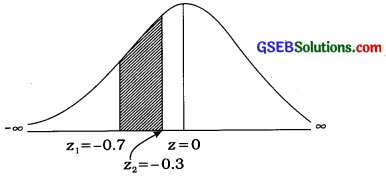P [40 ≤ X ≤ 60]
= P[- 0.7 ≤ Z ≤ – 0.3]
= [- 0.7 ≤ Z ≤ 0] – P [- 0.3 ≤ Z ≤ 0]
= 0.2580 – 0.1179
= 0.1401Question 8.
A normal variable X has following probability density function:
f(x) = constant ∙ $$e^{-\frac{1}{200}(x-50)^{2}}$$, – ∞o < x < ∞
From this distribution, answer the following questions:
( 1) Find median.
(2) Find estimated values of the extreme quartiles.
(3) Find approximate value of quartile deviation.
(4) Find approximate value of mean deviation.
Here,
f(x) = constant × $$e^{-\frac{1}{200}(x-50)^{2}}$$, – ∞ < x < ∞
= constant × $$e^{-\frac{1}{2}}\left(\frac{x-50}{10}\right)^{2}$$, – ∞ < x < ∞
∴ µ = 50, σ = 10

(1) Median :
M = µ = 50

(2) Extreme quartiles Q1 and Q3:
25 % observations are less than Q1 and 25 % of observations are more than Q3.
∴ P [X ≤ Q1 = 0.25 and P [X ≥ Q3] = 0.25
z1 = $$\frac{Q_{1}-\mu}{\sigma}$$ = $$\frac{Q_{1}-50}{10}$$ and
z2 = $$\frac{Q_{3}-\mu}{\sigma}$$ = $$\frac{Q_{3}-50}{10}$$P[Z ≤ z1] = 0.25 and P [Z ≥ z2] = 0.25
∴ P [z1 ≤ Z ≤ 0] = P [- ∞ < Z ≤ 0] – P [- ∞ < Z ≤ z1]
= 0.50 – 0.25
= 0.25

and P [0 ≤ Z ≤ z2] = P [0 ≤ Z < ∞] – P [z2 ≤ Z < ∞]
= 0.50 – 0.25
= 0.25

For area 0.2486,
z1 = – 0.67 and z2 = 0.68
For area 0.2518,
z1 = – 0.68 and z2 = 0.68
∴ For area 0.25,
z1 = $$\frac{-0.67-0.68}{2}$$ = – 0.675 and
z2 = $$\frac{0.67+0.68}{2}$$ = – 0.675 and
Now, z1 = $$\frac{Q_{1}-50}{10}$$
∴ – 0.675 = $$\frac{Q_{1}-50}{10}$$
∴ – 0.675 × 10 = Q1 – 50
∴ – 6.75 = Q1 – 50
∴ Q1 = 50 – 6.75
∴ Q1 = 43.25

and z2 = $$\frac{Q_{3}-50}{10}$$
∴ 0.675 = $$\frac{Q_{3}-50}{10}$$
∴ 0.675 × 10 = Q3 – 50
∴ 6.75 = Q3 – 50
∴ Q3 = 50 + 6.75
∴ Q3 = 43.25
Hence, the extreme quartiles Q1 and Q3 obtained are 43.25 and 56.75 respectively.

(3) Quartile deviation :
In normal distribution,
Quartile deviation ≈ $$\frac{2}{3}$$σ
Putting σ = 10,
Quartile deviation ≈ $$\frac{2}{3}$$ × 10 ≈ $$\frac{20}{3}$$ ≈ 6.67

(4) Mean deviation:
In normal distribution,
Mean deviation ≈ $$\frac{4}{5}$$σ
Putting σ = 10,
Quartile deviation ≈ $$\frac{4}{5}$$ × 10
≈ 8